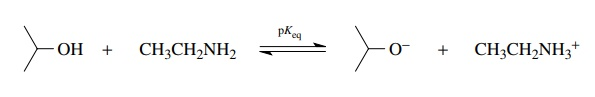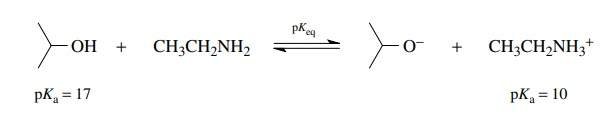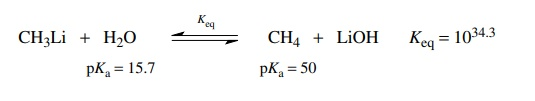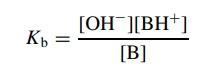# Acid-Base Equilibria

| Home | | Organic Chemistry |

## Chapter: Organic Chemistry : Acidity and Basicity

Since pKa values always refer to the ionization of an acid in water under standard conditions (dilute aqueous solution at 25◦ C), they can be used to predict the positions of acid–base equilibria.

ACID–BASE EQUILIBRIA

Since pKa values always refer to the ionization of an acid in water under standard conditions (dilute aqueous solution at 25 C), they can be used to predict the positions of acid–base equilibria. The position of the following equilibrium (pKeq) can be calculated by noting that in going from left to right isopropanol is acting as an acid.In going in the reverse direction, from right to left, the ethyl ammonium ion is acting as an acid. Having identified the compounds acting as acids on either side of the equilibrium, the pKa values for those acids are found and added to the equation.To evaluate the position of the equilibrium, pKeq is determined by the equation pKeq = pKa (reactant acid) pK a (product acid). For the above example pKeq = 17 10 = 7, and based on the definition of pK , Keq = 107 for this equi-librium. Thus the equilibrium lies far to the reactant side; that is, very little isopropoxide ion or ethyl ammonium ion is present at equilibrium. This tech-nique is applicable for virtually any acid–base equilibrium. The three required steps are to (a) write a balanced equation that describes the equilibrium to be analyzed, (b) identify the species which is acting as an acid on each side of the equilibrium and write down its pKa, and (c) subtract the pKa of the prod-uct acid from the pKa of the reactant acid to give the pK eq for the equilibrium in question. It is a requirement that the pKa’s of the acids on each side of the equilibrium are known or can be estimated reasonably well. Furthermore, the pKeq that is determined refers to the equilibrium in the direction it is writ-ten. It is therefore important to write the chemical equilibrium as you wish to analyze it.

The reaction of methyl lithium with water is an acid–base reaction. Going from left to right, water donates a proton to CH3Li so it functions as the acid on the left. Going from right to left, methane donates a proton to LiOH so it functions as the acid on the right. The pKa’s of the acids on either side of the equilibrium reaction are subtracted and pKeq = −34.3. Thus the equilibrium constant is Keq = 1034.3, which shows that the equilibrium lies very far to the right—so far to the right that for all practical purposes the conversion is quantitative.It is now common experimental practice to react ketones with lithium diiso-propyl amide (LDA) in order to generate the enolate of the ketone. This methodol-ogy has largely replaced the older approach to enolates, which employed alkoxide bases to remove a proton alpha to the carbonyl group. Comparison of the equilib-rium constants for these two acid–base reactions reveals why the LDA method is preferable. The use of the amide base leads to essentially complete conversion of the ketone to its enolate (Keq 1016). At equilibrium, there is virtually no unreacted ketone present in solution, only diisopropyl amine and the enolate, which can subsequently react cleanly and controllably with electrophiles which are added to the reaction mixture. In contrast, the use of an alkoxide base results in only partial conversion of the ketone to its enolate (Keq 102) so that at equilibrium the enolate, even greater amounts of the unreacted ketone, and some unreacted alkoxide are present in the reaction mixture. It is therefore difficult to react the enolate with an added electrophile while avoiding competing reac-tions of the enolate with the unreacted ketone and the alkoxide with the added electrophile. Thus control of the reaction is difficult and consequently yields are decreased and the product mixture is more complex.The ability to make good estimates of acid–base equilibrium constants is an invaluable aid in thinking about organic reactions and processes. Moreover, experimental workup procedures often require pH control that can be easily understood on the basis of pKa considerations. Thus the concept of acid strength is exceedingly important and should be mastered.

A similar development can be used for the quantitation and comparison of the base strengths of organic bases (the ability to accept protons from acids). To do this, Kb is defined asthe fraction of organic base which removes a proton from the standard acid—water.

A scale of Kb’s was developed with which to compare base strengths quantitatively.

The situation can become quite confusing since two sets of constants are defined, Ka and Kb. It turns out that acidities and basicities are inversely related by the ionization constant of water. That is, the stronger an acid is in donating a proton to water, the weaker its conjugate base is in removing a proton from water. This seems eminently reasonable since if something gives up a proton easily, then it should not take protons back easily. Put in more chemical terms, strong acids have weak conjugate bases, and weak acids have strong conjugate bases. It has become the convention to list only pKa’s as a measure of both acidity and basicity. The only thing to remember is to assign the appropriate Ka to the reaction in the “acidic” direction.

For example, the base strengths of NH3 and CH3O can be compared in two different ways. First, the reactions of these two bases with water can be written as follows, and by the preceding analysis the two equilibrium constants can be estimated by identifying the acids on either sides of the two equilibria and subtracting their pKa’s.It is seen that the equilibrium for the reaction of methoxide with water lies much farther to the right (Keq = 10) than the reaction of ammonia with water (Keq = 106). Clearly methoxide is much better at removing a proton from water than is ammonia by about 10 . Therefore methoxide is a stronger base by about 107 than ammonia.

Alternatively it is noted that the conjugate acids of ammonia and methoxide are the ammonium ion and methanol, respectively, and the equations for their ionization in water areComparing these two equilibria, it is seen that methanol is a much weaker acid than ammonium ion by about 107 (i.e., the pKa of methanol is larger by about seven logarithm units than the pKa of ammonium); thus its conjugate base methoxide should be a much stronger base than ammonia by 107. Either method of analyzing the situation is acceptable, and each gives the same relative basicities without the need for two sets of ionization constants, Ka and Kb.